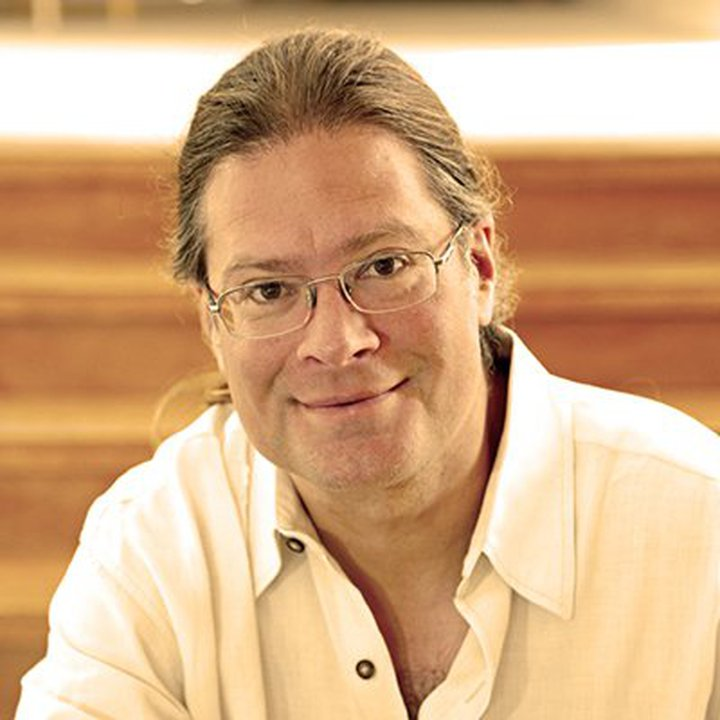Thaw Library - Miller Campus
Working Group

All day

Our campus is closed to the public for this event.

Over the past three decades, a bridge has been growing between computer science and statistical physics. Problems which are computationally hard—not just in the worst case, but even when the problem is randomly constructed—seem to be related to “glassy” systems in physics with many local optima and barriers between them. Many problems are conjectured to have a phase transition, sometimes called a statistical-computational gap, where their computational complexity jumps from polynomial to exponential. Moreover, this transition often occurs precisely where physics predicts the appearance of an energy barrier that separates accurate solutions from most of the state space. This includes problems in high-dimensional statistics and Bayesian inference such as noisy matrix and tensor factorization. The amount of noise plays the role of a temperature; when it crosses this threshold, our standard algorithms—spectral, Monte Carlo, belief propagation, and so on—all appear to fail, forcing the solver to perform an exhaustive search.

This working group will explore recent mathematical connections between spin glass theory and theoretical computer science, and explore how we can make these connections mathematically rigorous, connecting the structure of the landscape with notions of hardness such as information-theoretic bounds, the overlap gap property, sum-of-squares lower bounds, the low-degree likelihood ratio, and other properties related to computational hardness.

#### OrganizerCris MooreProfessor and Science Board Member at the Santa Fe Institute